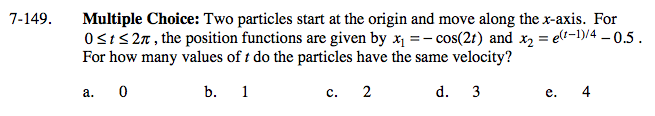### Home > CALC > Chapter 7 > Lesson 7.3.4 > Problem7-149

7-149.
1. Multiple Choice: Two particles start at the origin and move along the x-axis. For 0 ≤ t ≤ 2π, the position functions are given by x1 = − cos(2t) and x2 = e(t−1)/4 − 0.5. For how many values of t do the particles have the same velocity? Homework Help ✎

1. 0

2. 1

3. 2

4. 3

5. 4How many ways can you make v1 = v2?